GFG App
Open AppBrowser
Continue

# Types of Statistical Series

### What is Statistical Series?

Arranging classified data in some logical order such as according to the time of occurrence, size, or some other measurable or non-measurable characteristics is known as Statistical Series. These series are prepared for the proper presentation of collected and classified data in an arranged manner. For example, if the data containing the weight of 50 students in a class is put in a systematic order, it will be known as a statistical series.

### Types of  Statistical Series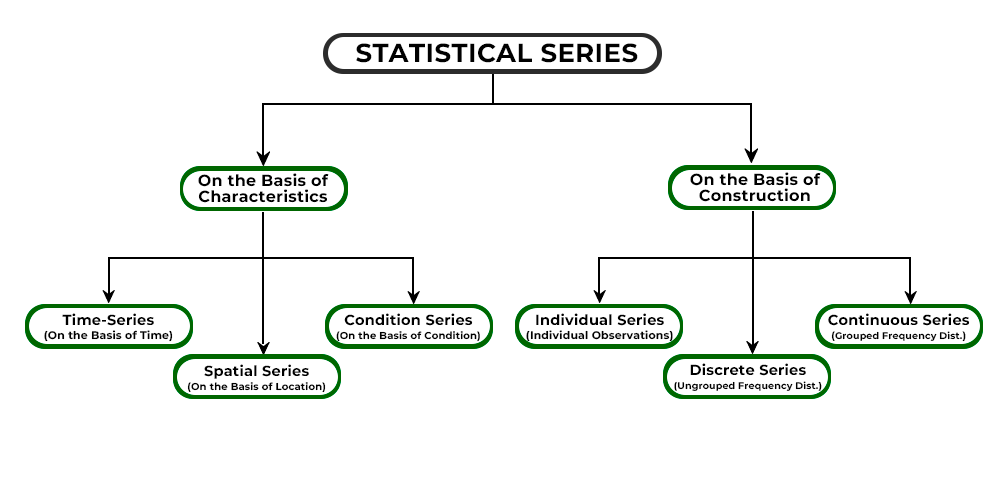Statistical Series can be classified On the Basis of Characteristics and On the Basis of Construction:

### A. On the Basis of Characteristics

When data is arranged based on the qualitative characteristics of the items, statistical series are of three kinds; viz., Time Series, Spatial Series, and Condition Series.

#### 1. Time Series:

If the different values taken by a variable in a period of time are arranged in chronological order, the series obtained is called a Time Series. Simply put, time series is a statistical series in which the given data is presented with regard to time unit; i.e., day, month, week, or year.

#### Example:

The following is the population of Delhi-NCR during the years 2010-2018.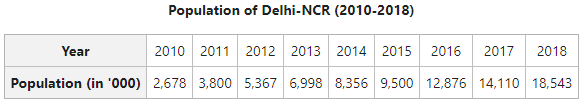It is a Time Series as the data is arranged according to the time unit; i.e., year.

#### 2. Spatial Series:

If the data is arranged according to the location or geographical consideration, then it is called a Spatial Series. The time factor remains constant in this kind of statistical series and the place changes.

#### Example:

The following is the sex ratio of 6 different states of India as per the Census of 2011.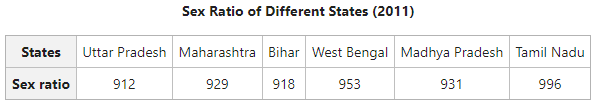In the above case, time is constant and the location of the data is changing; therefore, it is a spatial series.

#### 3. Condition Series:

If the data of a series is classified according to the changes occurring under certain conditions, then it is called a Condition Series.

#### Example:

The following is the table showing the arrangement of 40 students in a class according to their age.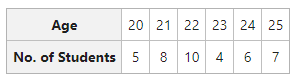It is a condition series because the data is arranged on basis of the age of the students

### B. On the Basis of Construction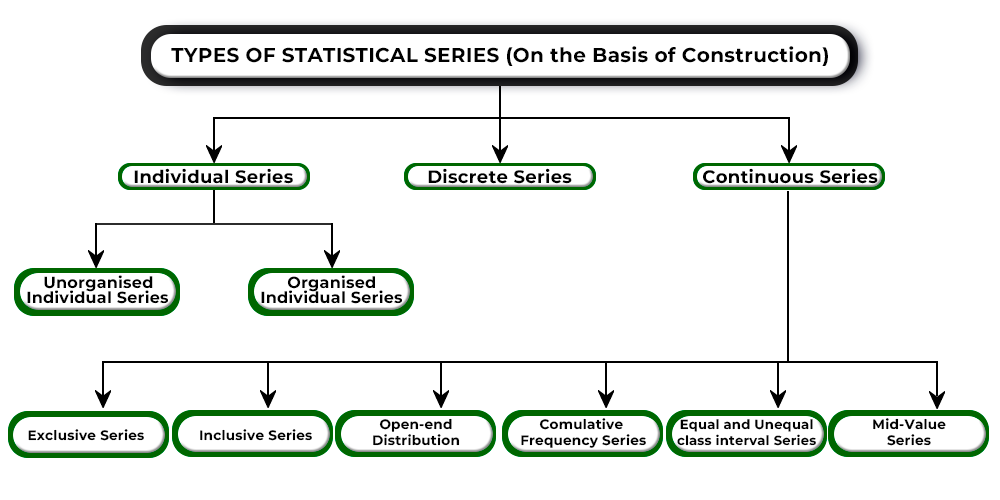Data can be arranged through statistical series on the basis of construction in the following types:

#### 1. Individual Series:

The series in which the items are listed singly is known as Individual Series. In simple terms, a separate value of the measurement is given to each item. For example, if the weight of 10 students of a class is given individually, then the resultant series will be an individual series. The two types of individual series are Unorganised Individual Series and Organised Individual Series.

i) Unorganised Individual Series: A series with raw data or an unarranged mass of data is known as Unorganised Series. Raw Data is the data in its original form. Simply put, when the investigator collects the data and has not arranged it in a systematic manner, then the collected data will be known as unorganised data. The data presented through unorganised series does not provide the investigator with any useful information; instead, it confuses them.

#### Example:

Marks obtained by 10 students in a class are given below: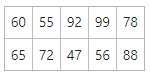The above table shows unorganised data as it is in its original or raw form.

ii) Organised Individual Series: A series with orderly arranged raw data is known as Organised Individual Series. There are two ways to present organised individual series; viz., According to Serial Number and According to Order of Magnitude (Ascending or Descending Order).

• According to Serial Number: An individual series can be arranged according to the serial number. For example, Marks obtained by 10 students arranged in the serial number is shown in the table below: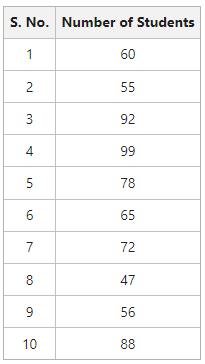• According to Order of Magnitude (Ascending or Descending Order): An individual series can be arranged in ascending or descending order. Arranging raw data in ascending or descending order of magnitude is known as Array, Arrays, Arraying of the data, or Arraying of the figures. For example, Marks obtained by 10 students arranged in ascending and descending order is shown in the table below: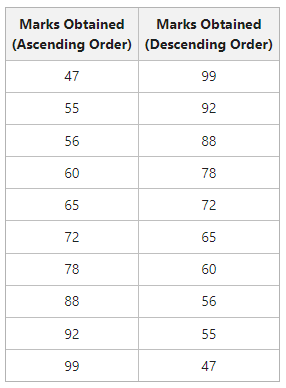#### 2. Discrete Series:

If the number of observations of a study is large, arranging data in individual series is not a solution. In that case, discrete series is a much better way to present the data. It is a series in which the individual values differ from each other by a definite amount. In this frequency distribution series, different values of the variables are shown with their respective frequencies. The classification of data for a discrete variable is known as Frequency Array. A discrete variable does not take a fractional value but takes just definite integral values.

#### How to Construct Discrete Frequency Distribution using Tally Marks

The method of Tally Bars or Tally Marks is used by the investigator to count the number of observations or the frequency of each value of the variable. The steps involved in the construction of discrete frequency distribution using tally marks are as follows:

1. Prepare a table with three columns with headings as Variable, Tally Marks, and Frequency.
2. The first column consists of each possible value of the variable.
3. The second column includes a tally mark denoted by | for every observation against its corresponding value. If a specific value has occurred for the fifth time, we put a cross on the tally mark (||||), by cutting the four tally bars and count the line cutting as the fifth number. For the sixth item, we will put another tally bar after leaving some space.
4. The third column includes the total of tally marks corresponding to each value of the variable.

#### Example:

Represent the marks of 22 students of a class in the form of discrete frequency distribution.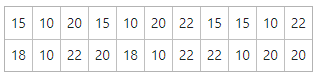#### Solution:

In the first column, the values written (marks) will be 10, 15, 18, 20, and 22. In the second column, the tally marks will be drawn according to the number of times they have occurred. Lastly, in the third column, the counted figure of the tally marks corresponding to the different values will be written. Thus, the frequency distribution table will be as follows: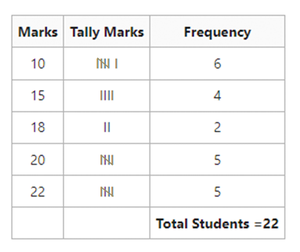From the above table, we can state that out of 22 students, 6 students got 10 marks, 4 students got 15 marks, 2 students got 18 marks, 5 students got 20 marks, and 5 students got 22 marks.

#### Relative Frequency Distribution:

Sometimes the actual frequencies are expressed as a percentage of total number of observations. In such cases, relative frequencies are obtained.

Example:

Transform the given data of marks obtained by 22 students of a class in a test in the form of discrete series. Also, calculate Relative Frequency.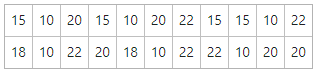Solution: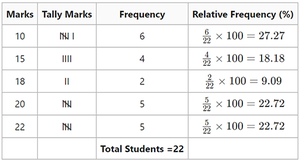#### 3. Continuous Series:

A discrete series cannot take any value in an interval; therefore, in cases where it is essential to represent continuous variables with a range of values of different items of a given data, Continuous Series is used. In this series, the measurements are only approximations and these approximations are expressed in the form of class intervals. The classes are formed from beginning to end, without any breaks.

Other names of Continuous Series are Frequency Distribution, Grouped Frequency Distribution, Series with Class Intervals, and Series of Grouped Data.

There are different types of continuous series; viz., Exclusive Series, Inclusive Series, Open-end Distribution, Cumulative Frequency Series, Equal and Unequal Class Interval Series, and Mid-value Series.

#### Important Terms under Continuous Series

The different terms used under Continuous Series are as follows:

i) Class: Class in Continuous Series refers to a group of numbers in which the items are placed. For example, 0-5, 5-10, 10-15, 15-20, 20-25, etc. It is essential for the investigator to clearly define the class so that they do not create any confusion. Besides, the classes should be exhaustive and mutually exclusive. The reason behind it is that it makes sure that a value of the variable comes in only one class and does not repeat.

ii) Number of Classes: The decision regarding the number of classes of a given data usually depends upon the judgement of the individual investigator. Even though there is no strict rule regarding the number of classes, the number should not be very small or very large and should be between 5 to 15. If the number of classes is less than 5, then each item of the data will be mentioned properly. In the same way, if the number of classes is more than 15, then it would become difficult and complicated in performing calculations.

iii) Class Limits: Class Limit is the lower and highest value of the variables within a class. In continuous series, the class limit is formed by the two numbers between which every class is located. The lowest value of the class is known as Lower Limit or l1; however, the highest value of the class is known as Upper Limit or l2. For example, if a class is 5-10, then 5 is the lower limit and 10 is the upper limit. If the lower limit of a class is either 0 or a multiple of 5, it becomes convenient for the investigator. Also, one should ensure that the class limits are whole numbers and that every item of the given data is included in those limits.

iv) Class Interval: It is the difference between the lower limit and upper limit of a class. The class interval of a series is usually indicated by i or c. Therefore, in symbolic terms,

Class Interval (i or c) = l2 – l1

Other names of Class Interval are Magnitude, Size, or Length of the class.

v) Width of Class Intervals: At the time of constructing the frequency distribution, it is suggested that the width of each class interval is equal in size. The formula for determining the size or width of each class interval is as follows:The following points should be taken into consideration before deciding on the width of various class intervals:

• Normally, a class interval of the series should be a multiple of 5. It is because grasping numbers like 5, 10, 15, etc., is easy.
• The width of the class interval should be such, which makes it convenient to determine the mid-value.
• The width of class intervals should be equal as far as possible and it makes it easy to represent graphically and analyse the data.
• A class’s observations should be uniformly distributed around its mid-value.

vi) Range: It is the difference between the lower limit of the first class interval and the upper limit of the last class interval. For example, if the classes of a distribution are 0-5, 5-10, 10-15, ………….till 45-50, then the range will be 50 – 0 = 50.

vii) Mid-Point or Mid-Value: It is the central point of a class interval. The formula to determine the Mid-Point of a class interval is as follows:For example, the mid-point of class interval 30-40 will beviii) Frequency: It is the number of items or observations that fall within a specific class interval. For example, if 20 students are there in a class (showing marks) 25-30, then the frequency will be 20. It means that there are 20 students who scored marks between 25-30.

ix) Frequency Distribution: A table showing how the different values of a variable are distributed in different classes along with their corresponding class frequencies, is known as frequency distribution. Simply put, it is a table which classifies the raw data of a quantitative variable in a comprehensive manner.

x) Class Frequency: It is the number of observations that corresponds to a specific class. It is also known as the Frequency of that Class and is denoted by f. The sum of all frequencies is known as N or ∑f. For example, if 20 students have scored marks between 30-40, then the class frequency will be 20.

#### Example:

From the marks given below of 30 students, prepare a frequency distribution table with classes 0-10, 10-20, and so on.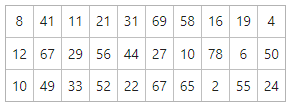#### Solution:

The steps to construct the frequency distribution table are as follows:

1. The first column of the table will include the class intervals starting from 0-10 till 60-70 as the value of the highest observation is 69.
2. The second column of the table will include the tally marks.
3. The third column of the table will include the frequency of the by counting the tally marks corresponding to the different values.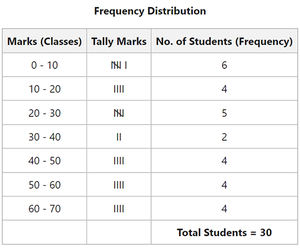My Personal Notes arrow_drop_up